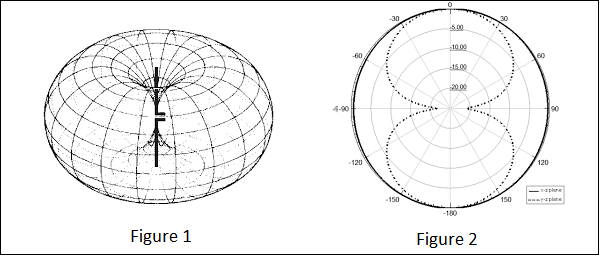# Antenna Theory - Isotropic Radiation

In the previous chapter, we have gone through the radiation pattern. To have a better analysis regarding the radiation of an antenna, a referential point is necessary. The radiation of an isotropic antenna, fills this space.

## Definition

Isotropic radiation is the radiation from a point source, radiating uniformly in all directions, with same intensity regardless of the direction of measurement.

The improvement of radiation pattern of an antenna is always assessed using the isotropic radiation of that antenna. If the radiation is equal in all directions, then it is known as isotropic radiation.

• The point source is an example of isotropic radiator. However, this isotropic radiation is practically impossible, because every antenna radiates its energy with some directivity.

• It has a doughnut-shaped pattern when viewed in 3D and a figure-of-eight pattern when viewed in 2D.The figures given above show the radiation pattern of an isotropic or Omni-directional pattern. Figure 1 illustrates the doughnut shaped pattern in 3D and Figure 2 illustrates the figure-of-eight pattern in 2D.

### Gain

The isotropic radiator has unity gain, which means having a gain factor of 1 in all directions. In terms of dB, it can be called as 0dB gain (zero loss).

According to the standard definition, “The amount of power that an isotropical antenna radiates to produce the peak power density observed in the direction of maximum antenna gain, is called as Equivalent Isotropic Radiated Power.”

If the radiated energy of an antenna is made to concentrate on one side or a particular direction, where the radiation is equivalent to that antenna’s isotropic radiated power, such a radiation would be termed as EIRP i.e. Equivalent Isotropic Radiated Power.

### Gain

Though isotropic radiation is an imaginary one, it is the best an antenna can give. The gain of such antenna will be 3dBi where 3dB is a factor of 2 and ‘i’ represents factor of isotropic condition.

If the radiation is focused in certain angle, then EIRP increases along with the antenna gain. Gain of the antenna is best achieved by focusing the antenna in certain direction.

$$ERP(dBW) = EIRP(dBW) - 2.15dBi$$